# Plus One Computer Science Chapter Wise Questions and Answers Chapter 4 Principles of Programming and Problem Solving

Students can Download Chapter 4 Principles of Programming and Problem Solving Questions and Answers, Plus One Computer Science Chapter Wise Questions and Answers helps you to revise the complete Kerala State Syllabus and score more marks in your examinations.

## Kerala Plus One Computer Science Chapter Wise Questions and Answers Chapter 4 Principles of Programming and Problem Solving

### Plus One Principles of Programming and Problem Solving One Mark Questions and Answers

Question 1.
The process of writing program is called _______.
programming or codingQuestion 2.
One who writes program is called _________
Programmer

Question 3.
The step by step procedure to solve a problem is known as ________
Algorithm

Question 4.
Diagrammatic representation of an algorithm is known as __________
Flow Chart

Question 5.
Program errors are known as _________
bugs

Question 6.
Process of detecting and correcting errors is called ________
debugging

Question 7.
Mr. Ramu represents an algorithm by using some symbols. This representation is called _______.
Flow Chart

Question 8.
(a) Parallelogram
(b) Rectangle
(c) Oval
(d) Rhombus
(c) OvalQuestion 9.
You are suffering from stomach ache. The doctor prescribes you for a scanning. This process is related in a phase in programming. Which phase is this?
Problem identification

Question 10.
Your friend asked you a doubt that to draw a flow chart parallelogram is used for what purpose?
To input/output

Question 11.
Mr. Anil wants to perform a multiplication which symbol is used to represent in a flow chart.
It is a processing so rectangle is used

Question 12.
Mr. George wants to check a number is greater than zero and to perform an operation while drawing a flow chart which symbol is used for this?
Rhombus

Question 13.
ANSI means ________
American National Standards Institute

Question 14.
To indicate the flow of an operation which symbol is used to draw a flow chart.

Question 15.
Mr. Johnson is drawing a flow chart but it is not fit in a single page. Which symbol will help him to complete the flow chart?
Connectors

Question 16.
Mr. Ravi developed a s/w student information system, He wants to protect the s/w from unauthorized copying. There is an act what is it?
Copy right actQuestion 17.
Odd man out.
(а) Parallelogram
(b) Oval
(c) Rectangle
(d) Star
(d) Star, others are flowchart symbols

Question 18.
Odd man out
(a) Oval
(b) Rhombus
(c) Connector
(d) Triangle
(d) Triangle, Others are flowchart symbols

Question 19.
Raju wrote a program and he wants to check the errors and correct if any? What process he has to do for this?
debugging

Question 20.
Odd one out.
(a) Problem identification
(b) Translation
(c) debugging
(d) Copy right, others are phases in programming

Question 21.
Odd man out.
(a) syntax error
(b) logical error
(c) Runtime error
(d) printer error
(d) Printer error, Others are different types of errors

Question 22.
A computerized system is not complete after the execution and testing phase? What is the next phase to complete the system?
Documentation.

Question 23.
Mr. Sathian takes a movie DVD from a CD library and he copies this into another DVD without permission. This process is called _______________
Piracy

Question 24.
Mr. Santhosh purchased a movie DVD and he takes several copies without permission. He is a _________
(a) Programmer
(c) Pirate
(d) Organizer
(c) Pirate

Question 25.
The symbol used for copy right is a _______
(a) @
(b) Copy
(c) &

Question 26.
Following are the advantages of flowcharts one among them is wrong. Find it.
(a) Better communication
(b) Effective analysis
(c) proper program documentation
(d) Modification easy
(d) Modification easy. It is a disadvantage.Question 27.
Which flow chart symbol has one entry flow and two exit flows?
Diamond

Question 28.
Which flow chart symbol is always used in pair?
connector

Question 29.
Program written in HLL is known as ____________
Source code

Question 30.
Some of the components in the phases of programming are given below. Write them in order of their occurrence. (1)

1. Translation
2. Documentation
3. Problem identification
4. Coding of a program

The chronological order is as follows

1. Problem Identification
2. Coding of a program
3. Translation
4. Documentation

Question 31.
_________ is the stage where programming errors are discovered and corrected.
Debugging or compiling

Question 32.
Ramesh has written a C++ program. During compilation and execution there were no errors. But he got a wrong output. Name the type of error he faced.
Logical Error

Question 33.
Pick out the software which rearranges the scattered files in the hard disk and improves the performance of the system.
(a) Backup software
(b) File compression software
(c) Disk defragmenter
(d) Antivirus software
(c) Disk defragmenter

Question 34.
Some phases in programming are given below.

1. Source coding
2. Execution
3. Translation
4. Problem study

These phases should follow a proper order. Choose the correct order from the following:
(a) 4 → 2 → 3 → 1
(b) 1 → 3 → 2 → 4
(c) 1 → 3 → 4 → 2
(d) 4 → 1 → 3 → 2
(d) 4 → 1 → 3 → 2

Question 35.
Which one of the following errors is identified at the time of compilation?
(a) Syntax error
(b) Logical error
(c) Run-time error
(d) All of these
(a) Syntax error

Question 36.
Pick the odd one out and give a reason for your findingc. This has one entry flow and more than one exit flow.

OR

b. Used for both input and output.

### Plus One Principles of Programming and Problem Solving Two Mark Questions and Answers

Question 1.
A debate on ‘Whether Free Software is to be promoted’ is planned in your class. You are asked to present points in support of Free Software. What would be your arguments, (at least three)?
Freedom to use Comparatively cheap Freedom to modify and redistributeQuestion 2.
Mr. Roy purchased a DVD of a movie and he found that on the cover there is a sentence copyright reserved and a mark ©. What is it? Briefly explain?
It is under the act of copyright and the trademark is © copyright is the property right that arises automatically when a person creates a new work by his own and by Law it prevents the others from the unauthorized or intentional copying of this without the permission of the creator.

Question 3.
Can a person who knows only Malayalam talk to a person who knows only Sanskrit normally consider the corresponding situation in a computer program and justify your answer?
Normally it is very difficult to communicate. But it is possible with the help of a translator. Translation is the process of converting programs written in High Level Language into Low Level Language (machine Language). The compiler or interpreter is used for this purpose. It is a program.

Question 4.
Define the term, debugging. Write the names of two phases that are included in debugging. (2)

OR

Define the different types of errors that are encountered during the compilation and running of a program.
Debugging:
The program errors are called ‘bugs’ and the process of detecting and correcting errors is called debugging. Compilation and running are the two phases.

OR

In general there are two types of errors syntax errors and logical errors. When the rules or syntax of the language are not followed then syntax errors occurred and it is displayed after compilation.

When the logic of a program is wrong then logical errors occurred and it is not displayed after compilation but it is displayed in the execution and testing phase.

Question 5.
Write an algorithm to input the scores obtained in three unit tests and find the average score.

OR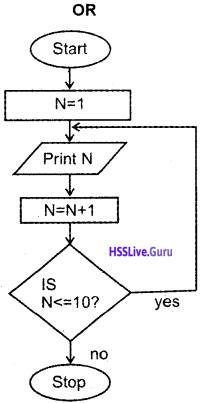Explain the flowchart and predict the output.

• Step 1: Start
• Step 2: Read S1, S2, S3
• Step 3: avg = S1 + S2 + S3/3
• Step 4: Print avg
• Step 5: Stop

OR

This flowchart is used to print the numbers as 1,2, 3, ………, 10.Question 6.
Differentiate between top down design and bottom up design in problem sloving.
Bottom up design:
Here also larger programs are divided into smaller ones and the smaller ones are again subdivided until the lowest level of detail has been reached. We start solving from the lowest module onwards. This approach is called Bottom up design.

Question 7.
Answer any one question from 5 (a) and 5 (b).
1. Draw a flowchart for the following algorithm.

• Step 1: Start
• Step 2: Input N
• Step 3: S = 0, K = 1
• Step 4: S = S + K
• Step 5: K = K + 1
• Step 6: If K < = N Then Go to Step 4
• Step 7: Print S
• Step 8: Stop

OR

2. Name the two stages in programming where debugging process is involved. What kinds of errors are removed in each of these stages?
1.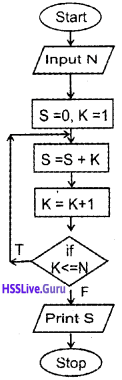2. The two stages are compile time and run time. In the debugging process can remove syntax error, logical error and runtime error.

Question 8.
Answer any one question from 7(1) and 7(2)
1. Observe the following portion of a flowchart. Fill in the blank symbols with proper instructions to get 321 as the output.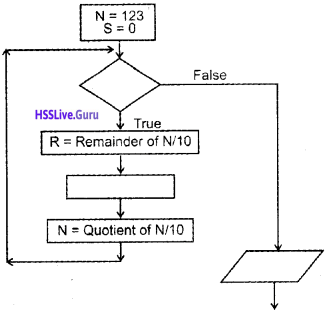2. The following flowchart can be used to print the numbers from 1 to 100. Identify another problem that can be solved using this flowchart and write the required instructions in the symbols.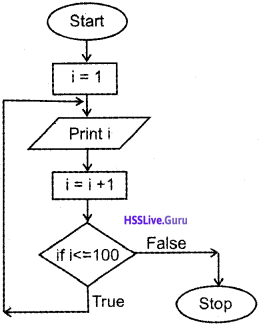1.2. The following flowchart can be used to store another problem such as used to print odd numbers lessthan 200.Question 9.
Write an algorithm to print the numbers upto 100 in reverse order, That is the output should be as 100, 99, 98, 97, …………., 1

OR

Draw a flow chart to check whether the given number is positive, negative or zero.

• Step 1: Start
• Step 2: Set i ← 100
• Step 3: if i <= 0 then go to step 6
• Step 4: Print i
• Step 5: Set i ← i — 1 go to step 3
• Step 6: Stop

OR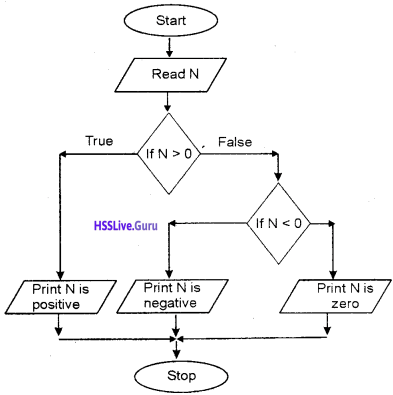### Plus One Principles of Programming and Problem Solving Three Mark Questions and Answers

Question 1.
When you try to execute a program, there are chances of errors at various stages, Mention the types of errors and explain.
1. Syntax error,
eg: 5 = x

2. Logic error:
If the programmer maks any logical mistakes, it is known as logical error.
eg: To find the sum of values A and B and store it in a variable C you have to write C = A + B. Instead of this if you write C = A × B, it is called logic error.

3. Runtime error:
An error occured at run time due to inappropriate data.
eg: To calculate A/B a person gives zero to B. There is an error called division by zero error during run time.

Question 2.
Following is a flow chart to find and display the largest among three numbers. Some steps are missing in the flowchart. Redraw the flow chart by adding necessary steps and specify its purpose. How can this flow chart be modified without using a fourth variable?Question 3.
A flow chart is given below.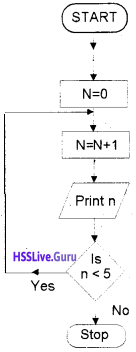1. What will be the output of the above flow chart?
2. How can you modify the above flow chart to display the even numbers upto 20, starting from 2.

1. 1, 2, 3, 4, 5

2.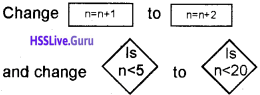Question 4.
Write an algorithm to check whether the given number is even or odd.

• Step 1: Start
• Step 2: Read a number to N
• Step 3: Divide the number by 2 and store the remainder in R.
• Step 4: If R = O Then go to Step 6
• Step 5: Print “N is odd” go to step 7
• Step 6: Print “N is even”
• Step 7: Stop

Question 5.
Write an algorithm to find the largest of 2 numbers?

• Step 1: Start
• Step 2: Input the values of A, B Compare A and B.
• Step 3: If A > B then go to step 5
• Step 4: Print “B is largest” go to Step 6
• Step 5: Print “A is largest”
• Step 6: Stop

Question 6.
Write an algorithm to find the sum of n natural numbers and average?

• step 1: Start
• Step 2: Set i ←1, S 0
• Step 3: Read a number and set to n
• Step 4: Computer i and n if i > n then go to step 7.
• Step 5: Set S ← S + i
• Step 6: i ← i + 1 go to step 4
• Step 7: avg ← S/n
• Step 8: Print “Sum = S and average = avg”
• Step 9: Stop

Question 7.
Write an algorithm to find the largest of 3 numbers.

• Step 1: Start
• Step 2: Read 3 numbers and store in A, B, C
• Step 3: Compare A and B. lf A > Bthengotostep 6
• Step 4: Compare B and C if C > B then go to step 8
• Step 5: print “B is largest” go to step 9
• Step 6: Compare A and C if C > A then go to step 8
• Step 7: Print”A is largest” go to step 9
• Step 8: Print “C is largest”
• Step 9: StopQuestion 8.
Write an algorithm to calculate the simple interest (I =P × N × R/100)

• Step 1: Start
• Step 2: Read 3 values for P, N, R
• Step 3: Calculate I ← P × N × R/100
• Step 4: Print “The simple interest = l”
• Step 5: Stop

Question 9.
Write an algorithm to calculate the compound interest (C.l = P × (1 + r/100)n – P)

• Step 1: Start
• Step 2: Read 3 number for p, n, r
• Step 3: Calculate C.I = p × (1 + r/100)n – p
• Step 4: Print “The compound Interest = C.l”
• Step 5: Stop

Question 10.
Write an algorithm to find the cube of first n natural numbers (eg: 1, 8, 27, …., n3)

• Step 1: Star
• Step 2: Set i ← 1
• Step 3: Read a number and store in n
• Step 4: Compare i and n if i > n then go to step 7
• Step 5: Print i × i × i
• Step 6: i ← i + 1 go to step 4
• Step 7: Stop

Question 11.
Write an algorithm to read a number and find its factorial (n ! = n × (n – 1) × (n – 2) ×………..3 × 2 × 1)

• Step 1: Start
• Step 2: Fact ← 1
• Step 3: Read a number and store in n
• Step 4: If n = 0 then go to step 7
• Step 5: Fact ← Fact × n
• Step 6: n ← n – 1 go to step 4
• Step 7: Print “Factorial is fact”
• Step 8: Stop

Question 12.
Draw a flow chart to find the sum of n natural numbers and average.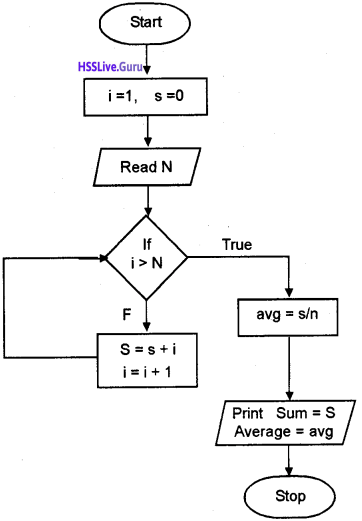Question 13.
Draw a flow chart to find the largest of 3 numbers.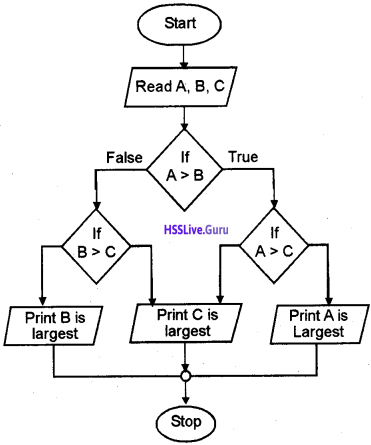Question 14.
Draw a flow chart to find the largest of 2 numbers.Question 15.
Draw a flow chart to check whether the given number is even or odd.Question 16.
Draw a flow chart to calculate simple interest.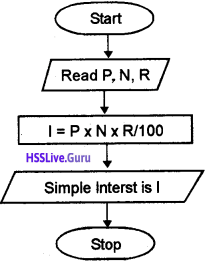Question 17.
Draw a flow chart to calculate compound interest.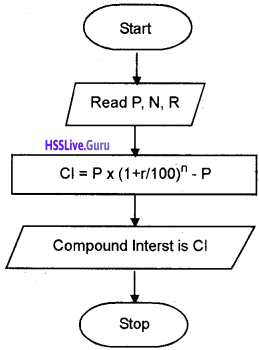Question 18.
Draw a flow chart to find the cube of n natural numbers.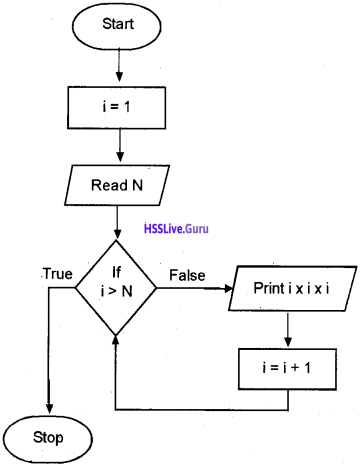Question 19.
Draw a flow chart to read a number and find its factorial.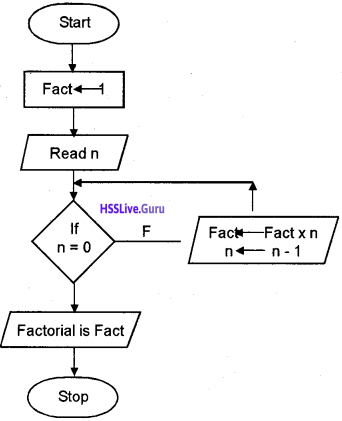Question 20.
Mr. Vimal wants to represent a problem by using a flowchart, which symbols are used for this. Explain.
Flow chart symbols are explained below
1. Terminal (Oval)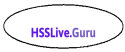It is used to indicate the beginning and ending of a problem

2. Input/Output (parallelogram)It is used to take input or print output.

3. Processing (Rectangle)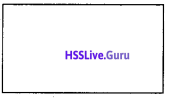It is used to represent processing. That means to represent arithmetic operation such as addition, subtraction, multiplication.

4. Decision (Rhombus)It is used to represent decision making. One exit path will be executed at a time.

5. Flowlines (Arrows)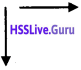It is used to represent the flow of operation

6. Connector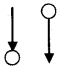These symbols will help us to complete the flow chart, which is not fit in a single page. A connector symbol is represented by a circle and a letter or digit is placed within the circle to indicate the link.

Question 21.
Jeena uses an algorithm to represent a problem while Neena uses flowchart which is better? Justify your answer?
Flowchart is better. The advantages of flow chart is given below.
1. Better communication:
A flow chart is a pictorial representation while an algorithm is a step by step procedure to solve a program. A programmer can easily explain the program logic using a flow chart.

2. Effective analysis:
The program can be analyzed effectively through the flow chart.

3. Effective synthesis:
If a problem is big it can be divided into small modules and the solution for each module is represented in flowchart separately and can be joined together final system design.

4. Proper program documentation:
A flow chart will help to create a document that will help the company in the absence of a programmer.

5. Efficient coding:
With the help of a flowchart it is easy to write program by using a computer language.Question 22.
A flow chart is a better method to represent a program. But it has some limitation what are they?
The limitations are given below

• To draw a flowchart, it is time consuming and laborious work.
• If any change or modification in the logic we may have to redraw a new flow chart.
• No standards to determine how much detail can include in a flow chart.

Question 23.

 1. Problem identification a. Flowchart 2. Steps to obtain. the solution b. Syntax Error 3. Coding c. Runtime Error 4. Translation d. COBOL 5. Debugging e. X-ray 6. Execution & Testing f. Compiler

1 – e
2 – a
3 – d
4 – f
5 – b
6 – c

Question 24.
Alvis executes an error free program but he got an error. Explain different types of error in detail.
There are two types of errors in a program before execution and testing phase.They are syntax error and logical error. When the programmer violates the rules or syntax of the programming language then the syntax error occurred.
eg: It involves incorrect punctuation.

Keywords are used for other purposes, violates the structure etc,… It detects the compiler and displays an error message that include the line number and give a clue of the nature of the error, When the programmer makes any mistakes in the logic, that types of errors are called logical error. It does not detect by the compiler but we will get a wrong output.

The program must be tested to check whether it is error free or not. The program must be tested by giving input test data and check whether it is right or wring with the known results. The third type of errors are Runtime errors.

This may be due to the in appropriate data while execution. For example consider B/C. If the end user gives a value zero for c, the execution will be interrupted because division by zero is not possible. These situation must be anticipated and must be handled.

Question 25.
The following are the phases in programming. The order is wrong rearrange them in correct order.

1. Debugging
2. Coding
3. Derive the steps to obtain the solution
4. Documentation
5. Translation
6. Problem identification
7. Execution and testing

The correct order is given below.

1. Problem identification
2. Derive the steps to obtain the solution
3. Coding
4. Translation
5. Debugging
6. Execution and testing
7. DocumentationQuestion 26.
Draw a flow chart to input ten different numbers and find their average.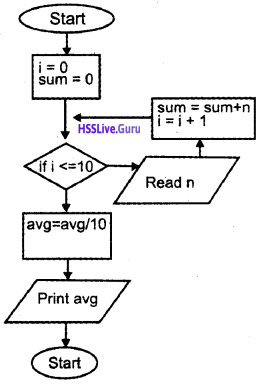Question 27.
Draw the flow chart to find the sum of first N natural numbers.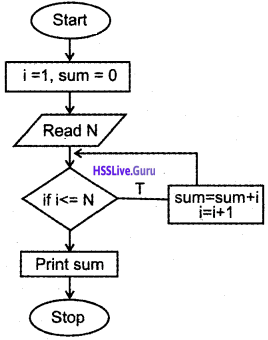Question 28.
Make a flow chart using the given labelled symbols, for finding the sum of all even numbers upto ‘N’

OR

Write an algorithm to accept an integer number and print the factors of it.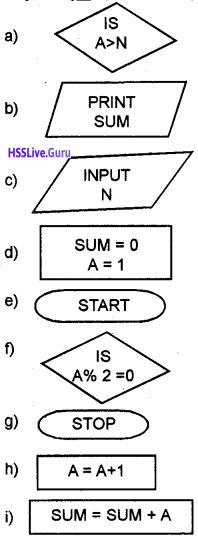Draw flowchart in any of the following order
e, c, d, f, i, h, a, b, g
e, d, c, f, i, h, a, b, g
e, c, d, a, f, i, h, b, g
e, d, c, a, f, i, h, b, g

• Step 1: Start
• Step 2: Input n
• Step 3: i = I
• Step 4: if i <= n/2 then repeat step 5 & 6
• step 5: if n % i == 0 print i
• Step 6: i = i + I
• Step 7: StopQuestion 29.
List the two approaches followed in problem solving or programming. How do they differ?
Approaches in problem solving:
(a) Top down design:
Larger programs are divided into smaller ones and solve each tasks by performing simpler activities. This concept is known as top down design in problem solving

(b) Bottom up design:
Here also larger programs are divided into smaller ones and the smaller ones are again subdivided until the lowest level of detail has been reached. We start solving from the lowest module onwards. This approach is called Bottom up design.

Phases in Programming
1. Problem identification:
This is the first phase in programming. The problem must be identified then only it can be solved, for this we may have to answer some questions.

During this phase we have to identify the data, its type, quantity and formula to be used as well as what activities are involved to get the desired out put is also identified for example if you are suffering from stomach ache and consult a Doctor.

To diagnose the disease the Doctor may ask you some question regarding the diet, duration of pain, previous occurrences etc, and examine some parts of your body by using stethoscope X-ray, scanning etc.

2. Deriving the steps to obtain the solution. There are two methods, Algorithm and flowchart, are used for this.
(a) Algorithm:
The step-by-step procedure to solve a problem is known as algorithm. It comes from the name of a famous Arab mathematician Abu Jafer Mohammed Ibn Musaa Al-Khowarizmi. The last part of his name Al-Khowarizmi was corrected to algorithm.

(b) Flowchart:
The pictorial or graphical representation of an algorithm is called flowchart.

Question 30.
Write an algorithm to find the sum of the squres of the digits of a number. (For example, if 235 is the input, the output should be 22 + 32 + 52 = 38)

• Step 1: Start
• Step 2: Set S = O
• Step 4: if N > O repeat step 5, 6 and 7
• Step 5: Find the remainder. That is rem = N % 10
• Step 6 : S = S + rem × rem
• Step 7 : N = N/10
• Step 8 : Print S
• Step 9 : Stop

Question 31.
Consider the following algorithm and answer the following questions:

• Step 1: Start
• Step 2 : N = 2, S = 0
• Step 3: Repeat Step 4, Step 5 while N <= 10
• Step 4: S = S + N
• Step 5: N = N + 2
• Step 6: Print S
• Step 7: Stop
1. Predict the output of the above algorithm.
2. Draw a flowchart for the above algorithm

1. The output is 30

2.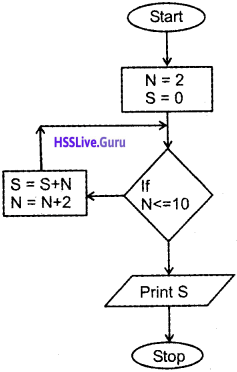Question 32.
“It is better to give proper documentation within the program”. Give a reason.
It is the last phase in programming. A computerized system must be documented properly and it is an ongoing process that starts in the first phase and continues till its implementation. It is helpful for the modification of the program later.

### Plus One Principles of Programming and Problem Solving Five Mark Questions and Answers

Question 1.
Mr. Arun wants to develop a program to computerize the functions of supermarket. Explain different phases he has to undergo is detail.

OR

Briefly explain different phases in programming.
The different phases in programming is given below:
1. Problem identification:
This is the first phase in programming. The problem must be identified then only it can be solved, for this we may have to answer some questions.
During this phase we have to identify the data, its type, quantity and formula to be used as well as what activities are involved to get the desired output is also identified for example if you are suffering from stomach ache and consult a Doctor.

To diagnose the disease the doctor may ask you some question regarding the diet, duration of pain, previous occurrences, etc and examine some parts of your body by using stethoscope X-ray, scanning, etc.

2. Deriving the steps to obtain the solution.
There are two methods, Algorithm and flowchart, are used for this.
(a) Algorithm:
The step-by-step procedure to solve a problem is known as algorithm. It comes from the name of a famous Arab mathematician Abu Jafer Mohammed Ibu Musaa Al-Khowarizmi. The last part of his name Al-Khowafizmi was corrected to algorithm.

(b) Flowchart:
The pictorial or graphical representation of an algorithm is called flowchart.

3. Coding:
The dummy codes (algorithm)or flow chart is converted into program by using a computer language such s Cobol, Pascal, C++, VB, Java, etc.

4. Translation:
The computer only knows machine language. It does not know HLL, but the human beings HLL is very easy to write programs. Therefore a translation is needed to convert a program written in HLL into machine code (object code).

During this step, the syntax errors of the program will be displayed. These errors are to be corrected and this process will be continued till we get “No errors” message. Then it is ready for execution.

5. Debugging:
The program errors are called ‘bugs’ and the process of detecting and correcting errors is called debugging. In general there are two types of errors syntax errors and logical errors. When the rules or syntax of the language are not followed then syntax errors occurred and it is displayed after compilation.

When the logic of a program is wrong then logical errors occurred and it is not displayed after compilation but it is displayed in the execution and testing phase.

6. Execution and Testing:
In this phase the program will be executed and give test data for testing the purpose of this is to determine whether the result produced by the program is correct or not. There is a chance of another type of error, Run time error, this may be due to inappropriate data.

7. Documentation:
It is the last phase in programming. A computerized system must be documented properly and it is an ongoing process that starts in the first phase and continues till its implementation. It is helpful for the modification of the program later.Question 2.
Briefly explain the characteristic of an algorithm.